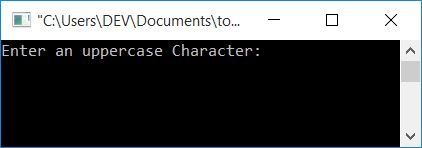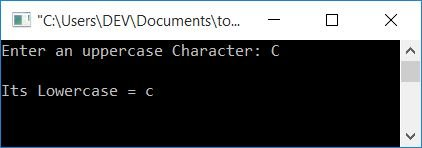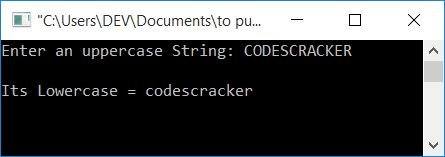# C Program to Convert Uppercase to Lowercase

In this article, you will learn how to convert a given character or string from uppercase to lowercase. Here is the list of programs that are available:

But before going to these programs, let's first discuss some of the main topics involved in them.

### Uppercase Character

Any character written in capital letters is known as an "uppercase" character. For example, C.

### Uppercase String

If each and every character of a string is written in capital letters, then that string can be called an "uppercase string." CODESCRACKER, for example.

### Lowercase Character

Any character written in small letters is known as a lowercase character. For example, c.

### Lowercase String

If each and every character of a string is written in small letters, then that string can be called a lowercase string. For example, codescracker.

### Uppercase to lowercase conversion formula

The formula to convert uppercase characters to lowercase is

`lowerChar = upperChar + 32`

because the ASCII values of A–Z are 65–90 and a–z are 97–122. That is, the ASCII value of A (capital A) is 65, whereas the ASCII value of A (small a) is 97. and the difference is 32. The difference is in that the ASCII value of lowercase a is 32 greater than the ASCII value of uppercase a. So to convert from uppercase characters to lowercase, we have added 32 to the upperChar variable (which holds uppercase characters). Now let's move on to the program.

## Uppercase character to lowercase in C

The question is: write a program in C that converts any given character (in uppercase) from the user (at run-time) to a lowercase character. The answer to this question is given below.

```#include<stdio.h>
#include<conio.h>
int main()
{
char upperChar, lowerChar;
int ascii;
printf("Enter an uppercase Character: ");
scanf("%c", &upperChar);
ascii = upperChar;
lowerChar = ascii+32;
printf("\nIts Lowercase = %c", lowerChar);
getch();
return 0;
}```

This program is compiled and executed under the Code::Blocks IDE. Here is the sample run:Now supply any character in uppercase (a capital letter), say C, and press the ENTER key to see the following output:If the user enters "C" as an uppercase character input, then C gets initialized to the upperChar variable. And the statement:

`ascii = upperChar;`

meaning the ASCII value of C, that is, 67, gets initialized to an ascii variable. And the statement:

`lowerChar = ascii+32;`

means that ascii+32 or 67+32 (depending on the value of ascii) or 99 is assigned to the lowerChar variable.And while printing the lowerChar as a character, we have used the %c format specifier that prints the character whose ASCII value is 99. That is C.

## In C, convert uppercase strings to lowercase

Now let's convert the string from uppercase to lowercase. To convert an uppercase to lowercase string, you have to ask the user to enter the string (in uppercase) to convert it into lowercase. To change an uppercase string into a lowercase string, just add 32 to each and every character of the given string, as shown in the following program.

The question is: write a program in C that converts any given string (in uppercase) to an equivalent string in lowercase. The answer to this question is given below:

```#include<stdio.h>
#include<conio.h>
int main()
{
char upperString="", lowerString="";
int i=0;
printf("Enter an uppercase String: ");
gets(upperString);
while(upperString[i]!='\0')
{
lowerString[i] = upperString[i]+32;
i++;
}
printf("\nIts Lowercase = %s", lowerString);
getch();
return 0;
}```

The snapshot given below shows a sample run of the above program:## In C, use the Library Function to convert an uppercase string to a lowercase string

The last program of this article uses the standard library function strlwr(), which takes a string as an argument and returns the same string in lowercase form. Let's have a look at the program given below:

```#include<stdio.h>
#include<conio.h>
#include<string.h>
int main()
{
char str;
printf("Enter uppercase String: ");
gets(str);
printf("\nIts Lowercase = %s", strlwr(str));
getch();
return 0;
}```

This program will produce the same output as the previous one.

#### The same program in different languages

C Quiz

« Previous Program Next Program »